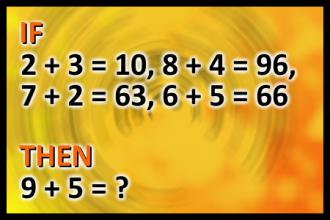BRAIN TEASERS# What is 9 + 5?

If 2+3=10, 8+4=96, 7+2=63, 6+5=66 then 9+5=?
The first user who solved this task is Eric Newton.
#brainteasers #math #riddles